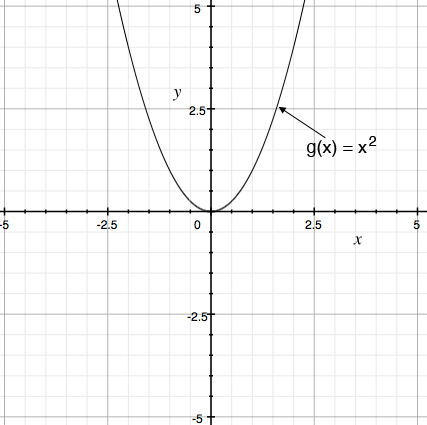SEARCH HOMEMath Central Quandaries & QueriesQuestion from Allison, a parent: Graph f(x)=x^2-4 and give the five point of the x/y intercepts.Hi Allison,

I assume you know how to graph $g(x) = x^2 .$For each value of $x$ the point on $f(x) = x^2 - 4$ is 4 units below the point with the same $x$ value on $g(x) = x^2$ so take the graph of $g(x)$ and move it down 4 units to obtain the graph of $f(x).$

I'm confused by second part of your question. I only see 3 intercepts not 5.

PennyMath Central is supported by the University of Regina and The Pacific Institute for the Mathematical Sciences.